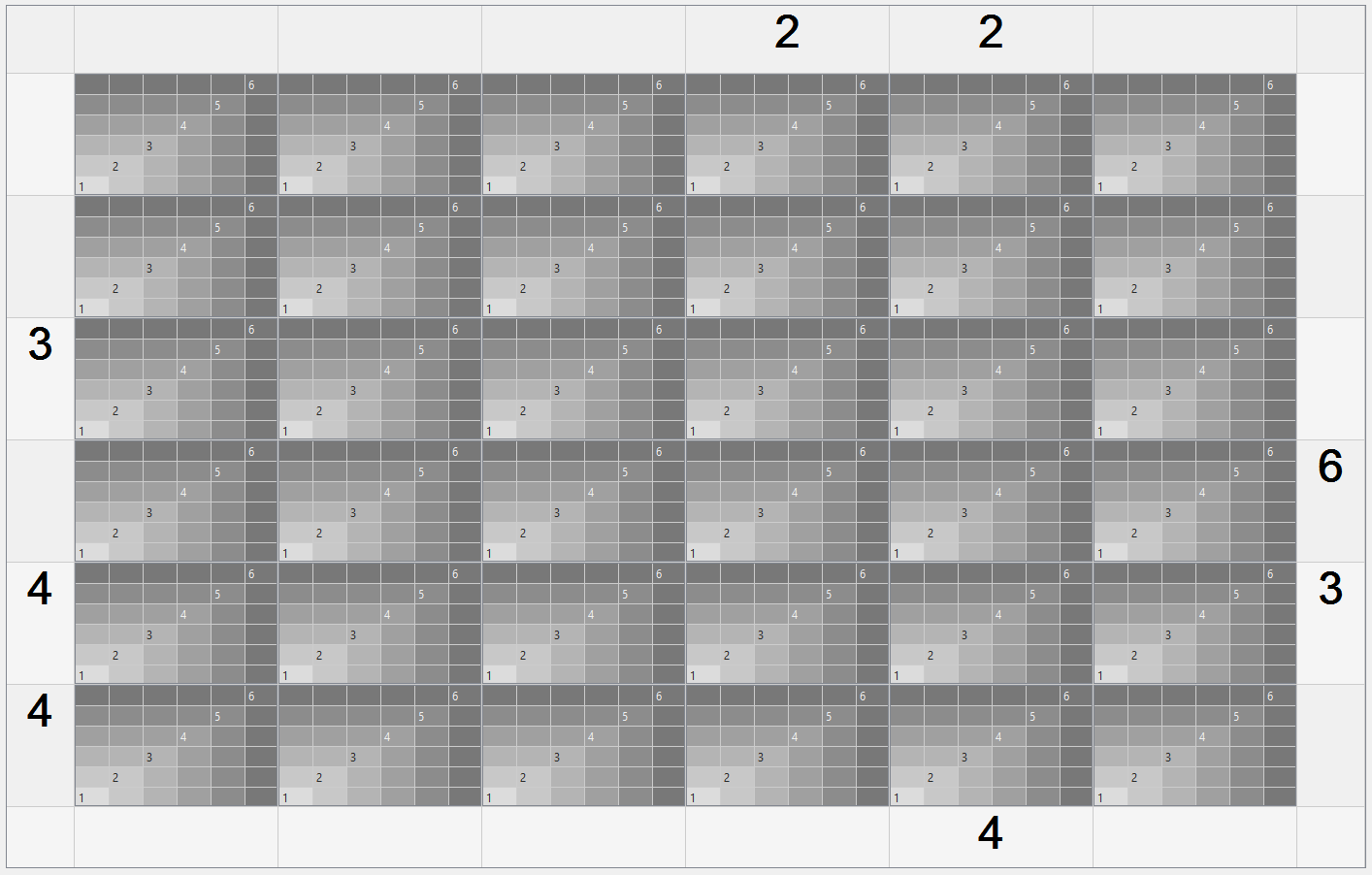# PySide2.QtWidgets.QTableWidget: why it's impossible to set column width less than 35 and row height less than 21?

• I am using code like following, trying to create a table of square cells. But when I try to use 5 (or even10) as cell size, I get table with rectangular cells with size of 35x21?

``````def create_square_table(self, cell_size=5, cell_number=5):
square_table = QtWidgets.QTableWidget()
square_table.setColumnCount(cell_number)
square_table.setRowCount(cell_number)
square_table.setHorizontalScrollBarPolicy(QtCore.Qt.ScrollBarAlwaysOff)
square_table.setVerticalScrollBarPolicy(QtCore.Qt.ScrollBarAlwaysOff)
square_table.setAlternatingRowColors(True)
square_table.setEnabled(False)

print('Column width:', square_table.columnWidth(0))
print('Row height:', square_table.rowHeight(0))
return square_table
table = self.create_square_table()
``````

The text printed in console is:

``````Column width: 35
Row height: 21
``````

• Hi and welcome to devnet,

What are you going to show in these little squares ?

• @SGaist , Hi! And thank you!
I want to print single digits (from 1 to 6) there.
Frankly speaking, I want to visualize the process of solving one kata from codewars.

• And how many do you want to show in total ?

I have the feeling that you might not be using the right tool (I may be wrong though).

• I want to show 6 by 6 tables, that will be CellWidgets of an outer table of 6 by 6. So small square cells are better for this task, because the whole table can be square and smaller than table that is made of rectanguler cells 35x21.

I was thinking about using gridLayot instead of tableWidgets. But in my opinion it will be more difficult to manage such an interface. Am I right?

This is how my intermediate result with 35x21 inner cells looks like:• I wonder if the Pixelator example would be a good starting point for you.

• Nice find @SGaist but man that is sure going to make that a lot more complicated -- I mean it would be nice if one could easily set the row/col size to what you want but I think that layout with those minimums looks okay as monitors do tend to wider than they are tall -- still I suppose its up to the designer on that